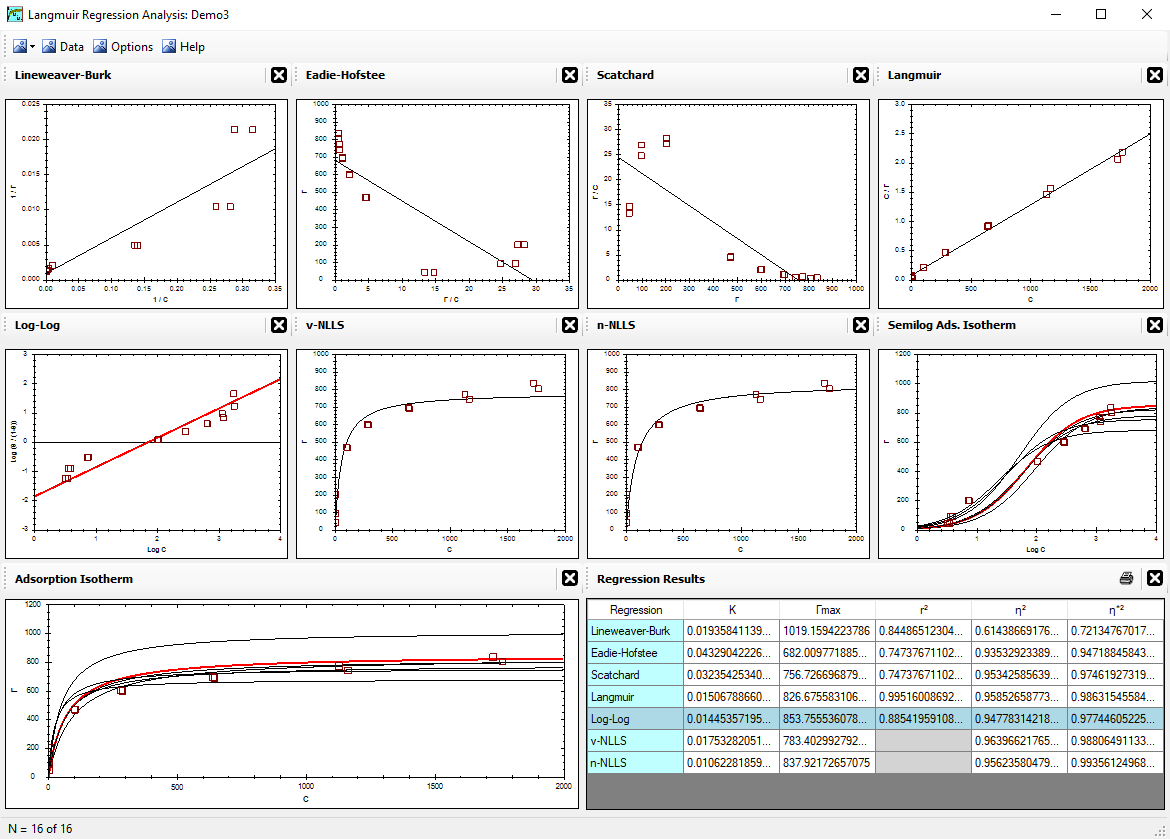Alfisol, Item 003: LMMpro, version 2.0 The Langmuir Optimization Program plus The Michaelis-Menten Optimization ProgramThe LangmuirEquation## The Langmuir Equation --- an overview of the Langmuir Isotherm.

The Langmuir Equation was introduced by Irwin Langmuir in 1916 to describe adsorption isotherms. He later presented data and a linear regression method for working with this equation in 1918. It was originally formulated for predicting the sorption of gases onto a solid phase. It is now also used to predict the sorption of aqueous compounds onto a solid phase. This is a mechanistic model that assumes that one reaction is involved in the sorption process, and that the distribution of the compounds between the two phases (gas-solid or liquid-solid) is controlled by an equilibrium constant. Langmuir received the Nobel Prize in Chemistry in 1932 for his work.

The Langmuir Equation assumes that the adsorption reaction involves a single reaction with a constant energy of adsorption. Using mass action laws for its derivation, these are the only assumptions made. Because of its simplicity, it is often the first theory tested on any adsorption data set.

Properly optimizing the parameters involved (namely Γmax and K) will greatly impact the conclusions made about the data collected. It may also impact the decisions made by the researcher as to which objective the current project should pursue next.

The equation can be derived as follows:

• Assume that only one reaction is involved, expressed as:
 S + A = SA
where S is the solid surface with nothing on it, A is the compound in the liquid or gaseous phase, and SA is the solid surface with compound A on it.
• Let c = (A) = equilibrium concentration of compound A in the liquid or gaseous phase, and define the equilibrium constant, K, as products over reactants:
 K = {SA} {S} c
• Define the total number of reactive sites, Γmax:
 Γmax = {SA} + {S}
• Combine the equations above in adsorption terms; that is, using {SA} terms:
 Γmax = {SA} + {SA} K c
• Finally, solve for {SA}, and let Γ = {SA}. The result is the Langmuir equation:
 Γ = Γmax K c 1 + K c

If K is known, if Γmax is known, and if the reaction is as described above, then the amount adsorbed (Γ) can be predicted for any concentration (c) of compound A. The two parameters (Γmax and K) are obtained by regression analysis of known c and Γ values.